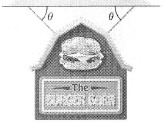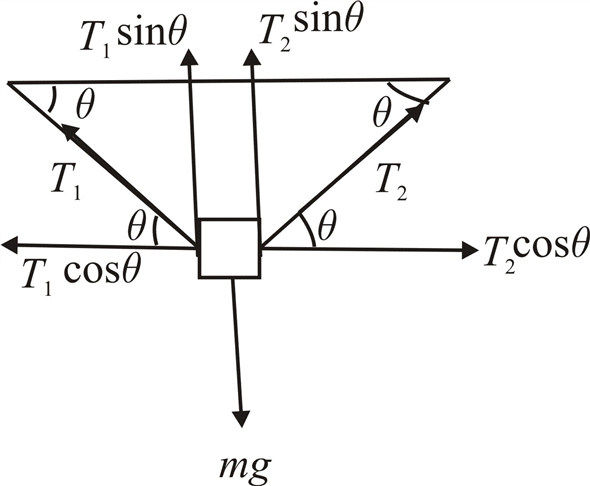Problem

# A store sign of mass 4.25 kg is hung by two wires that each make an angle of θ = 42.4° wit...

A store sign of mass 4.25 kg is hung by two wires that each make an angle of θ = 42.4° with the ceiling.What is the tension in each Wire?#### Step-by-Step Solution

Solution 1

Think:

Resolving the tension in each wire into horizontal and vertical components and we calculate the net force along x axis and y axis using Newton’s second law to obtain the tension in each wire.

Research:

The weight of the sign board will be directed downwards, tension in the wire will be resolved in to $$T \sin \theta$$ and $$T \cos \theta$$. Equating the forces along $$x$$ axis and $$y$$ axis we can evaluate the tension value.

Sketch:

The free body diagram for the system is shown below,Simplify:

Given data

Mass of the sign board is, $$m=4.25 \mathrm{~kg}$$

Angle made by the each wire with the ceiling is, $$\theta=42.4^{\circ}$$

Let the tensions in the two wires be $$T_{1}, T_{2}$$.

Using the free body diagram, we can calculate the net force along $$x$$ axis as,

\begin{aligned} \sum F_{x} &=m a_{x} \\ T_{1} \cos \theta-T_{2} \cos \theta &=0 \\ \left(T_{1}-T_{2}\right) \cos \theta &=0 \\ T_{1}-T_{2} &=0 \\ T_{1}=T_{2} \cdots \cdots(1) \end{aligned}

Similarly, we calculate the net force along $$y$$ axis as,

$$\begin{gathered} \sum F_{y}=m a_{y} \\ T_{1} \sin \theta+T_{2} \sin \theta-m g=0 \\ \sin \theta\left(T_{1}+T_{2}\right)=m g \\ T_{1}+T_{2}=\frac{m g}{\sin \theta} \ldots \ldots \text { (2) } \end{gathered}$$

Calculate:

Using the relation in equation (1), it will be easier to simplify the problem and the value of tension can be obtained.

Taking equation (2) for further simplification,

\begin{aligned} T_{1}+T_{2} &=\frac{m g}{\sin \theta} \\ 2 T_{1} &=\frac{m g}{\sin \theta} \quad\left[\text { Since }, T_{1}=T_{2}\right] \\ T_{1} &=\frac{(4.25 \mathrm{~kg})\left(9.81 \mathrm{~m} / \mathrm{s}^{2}\right)}{2\left(\sin 42.4^{\circ}\right)} \\ &=30.9 \mathrm{~N} \end{aligned}

Thus, the tension in each wire is, $$30.9 \mathrm{~N}$$